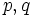# Classification of nilpotency-forcing numbers

## Statement

The following are equivalent for a natural number$n$:

1. Every group of order$n$ is a nilpotent group (and in particular, a finite nilpotent group)
2.$n$ has prime factorization of the form$n = p_1^{k_1}p_2^{k_2} \dots p_r^{k_r}$ where$p_i$ does not divide$p_j^l - 1$ for any$1 \le l \le k_j$ and$1 \le i,j \le r$.

The result is sometimes also called Schmidt's theorem. It is a corollary of the Schmidt-Iwasawa theorem.

## Facts used

1. Lagrange's theorem
2. Schmidt-Iwasawa theorem: This states that any group all of whose proper subgroups are nilpotent must be solvable; in particular, it must have a nilpotent maximal normal subgroup such that the quotient group is of prime order.
3. Equivalence of definitions of finite nilpotent group
4. Characteristic of normal implies normal

## Proof

### (1) implies (2)

It suffices to prove the contrapositive. In other words, we show that if the condition in (2) is violated, then we can construct a non-nilpotent group of that order.

Given: A natural number$n$, distinct primes$p,q$ and a natural number$l$ such that$pq^l$ divides$n$, and$p$ divides$q^l - 1$.

To prove: There is a non-nilpotent group of order$n$

Proof: Note that the product$pq^l$ divides$n$.

We can construct a non-nilpotent group$H$ of order$pq^l$ as follows: consider the additive group of the field of size$q^l$. The multiplicative group of this field is a cyclic group of size$q^l - 1$. Since$p$ divides$q^l - 1$, it has a subgroup of order$p$. Construct a semidirect product of the additive group of order$q^l$ with this subgroup of order$p$.

We can now construct a non-nilpotent group$G$ of order$n$ as the external direct product:$G = H \times \mathbb{Z}/(n/pq^l)\mathbb{Z}$

### (2) implies (1)

We prove the claim by induction on the number. Note that any divisor of a number that satisfies the condition also satisfies the condition.

Base case for induction ($n = 1$): Obvious

Inductive step: The inductive hypothesis is that the result holds for all smaller numbers.

Given: A natural number$n$ has prime factorization of the form$n = p_1^{k_1}p_2^{k_2} \dots p_r^{k_r}$ where$p_i$ does not divide$p_j^l - 1$ for any$1 \le l \le k_j$ and$1 \le i,j \le r$.$G$ is a group of order$n$.

To prove:$G$ is nilpotent

Proof:

Step no. Assertion/construction Facts used Give data used Previous steps used Explanation
1 Every proper subgroup of$G$ is nilpotent Fact (1) inductive hypothesis
arithmetic condition on$n$
Any divisor of$n$ satisfies the conditions necessary for the inductive hypothesis to apply. Applying the inductive hypothesis, we obtain that every subgroup is nilpotent.
2 Either$G$ is nilpotent or it contains a nilpotent maximal normal subgroup$N$ such that the quotient group$G/N$ is cyclic of prime order$p$ for some$p$ dividing$n$ Fact (2) Step (1) Step-fact combination direct
3$G$ has a nilpotent normal$p$-complement$K$. Facts (3), (4) Step (2)$G$ has a nilpotent normal subgroup of index$p$. This in turn has normal Sylow subgroups for all primes dividing its order, which are characteristic in it and therefore also normal in$G$. For all primes other than$p$, order considerations make these Sylow subgroups of$G$ as well.
4 Suppose$P$ is a$p$-Sylow subgroup of$G$. Then, every element of$P$ centralizes all elements of$K$. Thus,$G$ is an internal direct product of$P$ and$K$, and is nilpotent.$p_i$ does not divide$p_j^l - 1$ for$1 \le l \le k_j$ Step (3) [SHOW MORE]